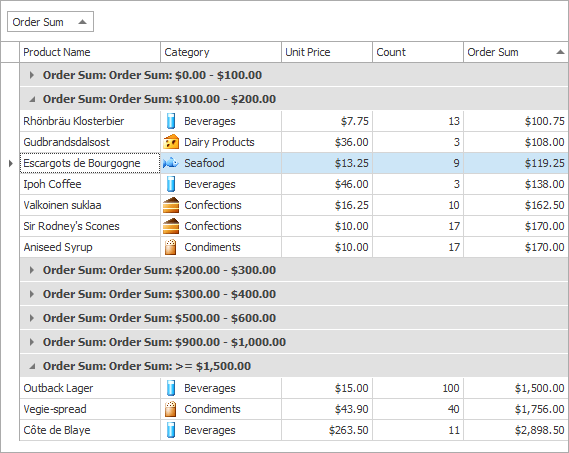# How to: Implement Custom Grouping

Assume that a Grid View’s “Order Sum” column contains numbers. When grouping is applied to this column, the rows which have values between 0 and 99 in this column should be combined into a single group, the rows whose values fall between 100 and 199 should be combined into another group, etc. To provide custom grouping logic the column’s GridColumn.SortMode property should be set to ColumnSortMode.Custom and the GridView.CustomColumnGroup event should be handled to implement the algorithm. In the following example the event’s Result parameter is set to 0 if the two rows being compared should be placed within the same group.

The ColumnView.CustomColumnDisplayText event is handled to replace the default text displayed within group rows.

The result of data being custom grouped is shown below:``````using DevExpress.XtraGrid;
using DevExpress.XtraGrid.Views.Base;
using DevExpress.XtraGrid.Views.Grid;
using DevExpress.XtraGrid.Views.Grid.ViewInfo;

// ...
gridView1.Columns["Order Sum"].SortMode = ColumnSortMode.Custom;

private void gridView1_CustomColumnGroup(object sender, CustomColumnSortEventArgs e) {
if(e.Column.FieldName == "Order Sum") {
double x = Math.Floor(Convert.ToDouble(e.Value1) / 100);
double y = Math.Floor(Convert.ToDouble(e.Value2) / 100);
int res = Comparer.Default.Compare(x, y);
if(x > 14 && y > 14) res = 0;
e.Result = res;
e.Handled = true;
}
}

private void gridView_CustomColumnDisplayText(object sender, DevExpress.XtraGrid.Views.Base.CustomColumnDisplayTextEventArgs e) {
GridView view = sender as GridView;
if(view == null) return;
if (e.Column.FieldName == "Order Sum" && e.IsForGroupRow) {
double rowValue = Convert.ToDouble(view.GetGroupRowValue(e.GroupRowHandle, e.Column));
double val = Math.Floor(rowValue / 100);
string groupRowInterval = string.Format("{0:c} - {1:c} ", val * 100, (val + 1) * 100);
if (val > 14)
groupRowInterval = string.Format(">= {0:c} ", val * 100);
e.DisplayText = "Order Sum: " + groupRowInterval;
}
}
``````